Introduction

The PointFore package can be loaded the usual way.

library(PointFore)

The main function is estimate.functional() which creates an object of class pointfore. Many parameters are available but most of them can be set to their default values. They are explained in details below through examples.

The function estimate.functional() provides a convenient wrapper for the estimation based on the gmm package. The main arguments are the forecast X and the realizations Y. Further, one can choose between quantiles and expectiles and the model that describes the forecasted level.

The available methods for a pointfore object are plot and summary.

Simulated Example

We illustrate the application of the package in a simple simulated example.

Generate data

First, we generate a time series Y with mean Y.mean. Further, we generate an optimal mean-forecast X.

set.seed(1)

Y.mean <- rnorm(200,0,1)

Y <- rnorm(200,Y.mean,1)

X <- Y.mean

Estimation of the functional

Now, we apply a class of constant expectile forecasts. The summary function shows the call and the estimation result, and the J-test of overidentifying restrictions.

res.const <- estimate.functional(
iden.fct = expectiles,
model = constant,
Y = Y,
X = X,
theta0 = 0.5)
#> Drop  1 case(s) because of chosen instruments

summary(res.const)
#> $call #> estimate.functional(iden.fct = expectiles, model = constant, #> theta0 = 0.5, Y = Y, X = X) #> #>$coefficients
#>           Estimate Std. Error  t value     Pr(>|t|)
#> Theta 0.4810124  0.0347885 13.82677 1.757478e-43
#>
#> $Jtest #> #> ## J-Test: degrees of freedom is 2 ## #> #> J-test P-value #> Test E(g)=0: 1.45544 0.48301 The results imply an expectile level close to 0.5. In a second step, we estimate a state-dependent expectile model, where the level of the expectile can depend on the current forecast. The linear probit model is already implemented in the PointFore package. The level of the forecast depends on the the state variable s, via the formula $level = inv.logit(\theta_1 + s \theta_2)= (1+exp(\theta_1 + s \theta_2)^{-1})^{-1}.$. res.flexible <- estimate.functional(iden.fct = expectiles, model = probit_linear, Y = Y,X = X, theta0 = c(0,0), stateVariable = X) #> Drop 1 case(s) because of chosen instruments summary(res.flexible) #>$call
#> estimate.functional(iden.fct = expectiles, model = probit_linear,
#>     theta0 = c(0, 0), Y = Y, X = X, stateVariable = X)
#>
#> $coefficients #> Estimate Std. Error t value Pr(>|t|) #> Theta -0.04812388 0.08743848 -0.5503742 0.5820628 #> Theta 0.03844594 0.08965164 0.4288370 0.6680418 #> #>$Jtest
#>
#>  ##  J-Test: degrees of freedom is 1  ##
#>
#>                 J-test   P-value
#> Test E(g)=0:    1.26840  0.26007

Plot results

For interpretation, the results can be plotted with the according pointwise confidence intervals. For state-dependent models the state is plotted on the x-axis. In green, we see a density estimate of the state.

plot(res.const)
plot(res.flexible)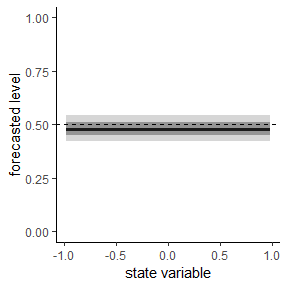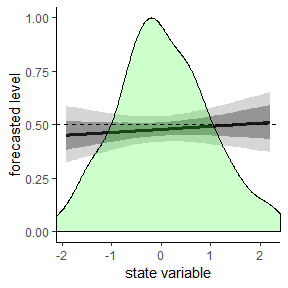Wald tests

It is straight forward to apply standard Wald tests to the estimated gmm-object. First, we have to load the car package.

library(car)

For the constant model, the mean forecast, which is equal to the $$0.5$$-expectile forecast cannot be rejected by a Wald-type test

linearHypothesis(res.const$gmm,"Theta=0.5") #> Linear hypothesis test #> #> Hypothesis: #> Theta = 0.5 #> #> Model 1: restricted model #> Model 2: res.const$gmm
#>
#>   Df  Chisq Pr(>Chisq)
#> 1
#> 2  1 0.2979     0.5852

Similarly, the flexible linear probit model cannot reject the mean-forecast ($$H_0: \theta_1=0 \wedge \theta_2 =0$$), nor state-independence($$H_0: \theta_2 =0$$).

linearHypothesis(res.flexible$gmm,c("Theta=0", "Theta=0")) #> Linear hypothesis test #> #> Hypothesis: #> Theta = 0 #> Theta = 0 #> #> Model 1: restricted model #> Model 2: res.flexible$gmm
#>
#>   Df  Chisq Pr(>Chisq)
#> 1
#> 2  2 0.4819     0.7859

linearHypothesis(res.flexible$gmm,"Theta=0") #> Linear hypothesis test #> #> Hypothesis: #> Theta = 0 #> #> Model 1: restricted model #> Model 2: res.flexible$gmm
#>
#>   Df  Chisq Pr(>Chisq)
#> 1
#> 2  1 0.1839      0.668

You can also create your own specification models, which describe how the levels of the quantile/expectile depend on the state variable.

The two parameters and are necessary. The output is the level and should always be in the unit interval.

break_model <- function(stateVariable, theta)
{
if(length(theta)!=2)
stop("Wrong dimension of theta")

return(boot::inv.logit(theta+theta*(stateVariable>0)))
}

Now, we can estimate the model

res.break <- estimate.functional(iden.fct = expectiles,
model = break_model,
Y = Y,X = X,
theta0 = c(0,0),
stateVariable = X)
#> Drop  1 case(s) because of chosen instruments

summary(res.break)
#> $call #> estimate.functional(iden.fct = expectiles, model = break_model, #> theta0 = c(0, 0), Y = Y, X = X, stateVariable = X) #> #>$coefficients
#>            Estimate Std. Error    t value  Pr(>|t|)
#> Theta -0.1410560  0.2081387 -0.6777018 0.4979608
#> Theta  0.1420977  0.3362443  0.4226025 0.6725853
#>
#> $Jtest #> #> ## J-Test: degrees of freedom is 1 ## #> #> J-test P-value #> Test E(g)=0: 1.27252 0.25929 plot(res.break)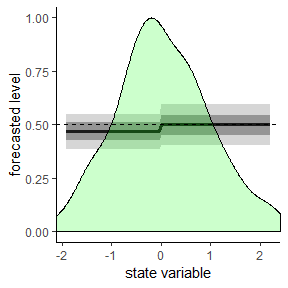If we use a forecast that is biased for positive means: X <- Y.mean + 0.2 *(Y.mean>0) res.break <- estimate.functional(iden.fct = expectiles, model = break_model, Y = Y,X = X, theta0 = c(0,0), stateVariable = X) #> Drop 1 case(s) because of chosen instruments summary(res.break) #>$call
#> estimate.functional(iden.fct = expectiles, model = break_model,
#>     theta0 = c(0, 0), Y = Y, X = X, stateVariable = X)
#>
#> $coefficients #> Estimate Std. Error t value Pr(>|t|) #> Theta -0.1440108 0.2067031 -0.6967034 0.48598846 #> Theta 0.7029084 0.3054015 2.3015878 0.02135843 #> #>$Jtest
#>
#>  ##  J-Test: degrees of freedom is 1  ##
#>
#>                 J-test   P-value
#> Test E(g)=0:    1.97414  0.16001

plot(res.break)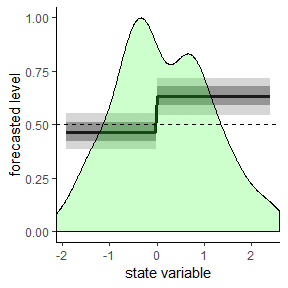A bias that can also be detected by the linear probit model, which has a similar shape.

res.flexible <- estimate.functional(iden.fct = expectiles,
model = probit_linear,
Y = Y,X = X,
theta0 = c(0,0),
stateVariable = X)
#> Drop  1 case(s) because of chosen instruments

summary(res.flexible)
#> $call #> estimate.functional(iden.fct = expectiles, model = probit_linear, #> theta0 = c(0, 0), Y = Y, X = X, stateVariable = X) #> #>$coefficients
#>            Estimate Std. Error  t value   Pr(>|t|)
#> Theta 0.09608041 0.09046600 1.062061 0.28820795
#> Theta 0.17283605 0.07911063 2.184739 0.02890801
#>
#> $Jtest #> #> ## J-Test: degrees of freedom is 1 ## #> #> J-test P-value #> Test E(g)=0: 1.99599 0.15772 plot(res.flexible)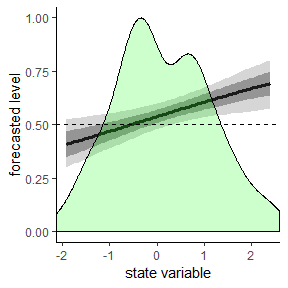Applying non-standard instruments However, the biased forecast is not optimal with respect to the linear probit model. The deviation can be detected by the J-test of overidentifying restrictions if we use appropriate instruments, that generate a sufficiently rich information set to test for. If we include the squared forecast with its original sign in the instruments, the test rejects optimality with respect to the linear probit model with a p-value below 0.05 and correctly cannot reject optimality with respect to the break model with a p-value of 0.11. res.flexible <- estimate.functional(iden.fct = expectiles, model = probit_linear, Y = Y,X = X, theta0 = c(0,0), instruments = c("lag(Y)","X","sign(X)*X^2"), stateVariable = X) #> Drop 1 case(s) because of chosen instruments summary(res.flexible)$Jtest
#>
#>  ##  J-Test: degrees of freedom is 2  ##
#>
#>                 J-test   P-value
#> Test E(g)=0:    8.28543  0.01588

res.break <- estimate.functional(iden.fct = expectiles,
model = probit_break,
Y = Y,X = X,
theta0 = c(0,0),
instruments = c("lag(Y)","X","sign(X)*X^2"),
stateVariable = X)
#> Drop  1 case(s) because of chosen instruments

summary(res.break)\$Jtest
#>
#>  ##  J-Test: degrees of freedom is 2  ##
#>
#>                 J-test  P-value
#> Test E(g)=0:    4.3678  0.1126